# A Multi-Channel Active Noise Control Algorithm Based on Variable Step Size

All sounds are mixed from a series of sound signals of different frequencies. If a sound can be artificially generated, the frequency of which is exactly the same as the noise to be eliminated, but the phase is opposite to that of the noise to be completely eliminated. Active noise control (ANC) is to add a circuit for noise analysis to the device, and through the controller to quickly calculate and analyze, generate an artificial sound signal that can cancel the external noise, and play the opposite phase signal through the speaker to cancel the target noise.

0 Preface

All sounds are mixed from a series of sound signals of different frequencies. If a sound can be artificially generated, the frequency of which is exactly the same as the noise to be eliminated, but the phase is opposite to that of the noise to be completely eliminated. Active noise control (ANC) is to add a circuit for noise analysis to the device, and through the controller to quickly calculate and analyze, generate an artificial sound signal that can cancel the external noise, and play the opposite phase signal through the speaker to cancel the target noise. ANC can be used in a variety of applications, including personal hearing devices[1-2]duct and room acoustic enhancement, engine exhaust noise suppression and improved vehicle enclosure wind noise, acoustics in aircraft cabins and vibrating machines[3-4]. With the rapid development of modern industry, the Power of most industrial equipment is getting bigger and bigger, the speed is getting faster and faster, and the noise hazard is becoming more and more prominent. Noise not only affects product quality, operation accuracy, shortens product life, endangers safety, but also pollutes the environment and affects human health. Therefore, mastering the noise control technology is a major issue facing the industrial development.

In practical research applications, the filtered x least mean square algorithm (filteredx LMS, FxLMS) The structure is simple and the algorithm is stable, so the FxLMS algorithm is the most widely used in ANC controllers; there is also an improved normalized LMSthe steady-state error can be reduced to a very small, but the convergence speed will be greatly affected; SVSLMS based on Sigmoid functionThe algorithm is also widely used, and its convergence speed is fast, but the steady-state error cannot meet the requirements.Such variable-step control algorithms[8-11] The main problem is that the convergence step size in the algorithm is mostly a function of the feedback error signal. After the iterative calculation of the filter, the convergence of the signal is not zero, which will cause the system to have a non-zero offset problem, which will easily lead to a large error after the system enters a steady state and no longer converges. Therefore, it has become a feasible direction to improve the noise reduction effect of low-frequency noise by constructing a variable-step active noise control system with the correlation function of the feedforward signal as the reference.

In the free field, the diameter of the “anechoic zone” produced by the single-channel active noise control system is approximately 1/10 the wavelength of the control sound, that is to say, the effective range of the conventional single-channel active control system is only twenty or thirty centimeters in diameter, which is far from enough for practical applications.Therefore, the active control of large area noise needs to use a multi-channel control algorithm to achieve[13-17]this paper proposes a multi-channel active control algorithm based on variable step size, and uses simulation experiments to verify its feasibility.

1 Control algorithm

1.1 Variable step size

When a fixed convergence step is used, the convergence accuracy and the convergence time of the system show an opposite situation, that is, when the convergence step is set smaller, the convergence accuracy is higher, but the convergence time is greatly prolonged; on the contrary, when the convergence step is set larger , the convergence time is reduced, but the convergence accuracy is greatly reduced. therefore,

In order to eliminate the limitation of fixed convergence step size FxLMS, ​​consider adopting variable convergence step size FxLMS algorithm.

In Variable Convergence Step Size Algorithm Based on Sigmiod Function (SVSLMS)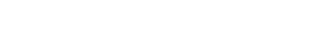From equation (1), the variable convergence step μ(n) is the Sigmiod function of e(n). During the initial convergence, the larger the error, the larger the value of the convergence factor; as the error gradually decreases, the value of the convergence factor also decreases. Therefore, the criteria of adaptive filtering are satisfied at the same time: fast convergence speed, tracking speed and small steady-state error. However, the calculation of this formula is cumbersome, and the stability of the error e(n) near 0 is not ideal, and it is easy to cause non-zero offset. In the normalized LMS algorithm, the variable step size is normalized with the power value of the reference input signal, so as to obtain the variable convergence step size μ related to the power of the reference input signal. The variable convergence factor function is expressed as: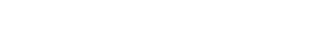Since the hardware implementation of the Euclidean square norm of x(n) is more complicated, and the reference input signal only takes the current instantaneous value, the Euclidean square norm of x(n) is equal to the square of the absolute value of x(n), which is improved as: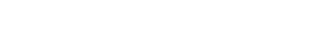where |x(n)|2 is the squared value of the instantaneous reference input signal in the nth iteration. It can be seen from equations (1) and (3) that in the calculation of the variable convergence factor, the operation of solving the autocorrelation matrix of the input vector is transformed into the square value operation of the scalar, which can improve the convergence, so let: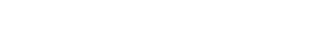In order to improve the problem of non-zero offset, change the input signal of variable step size to the variation of the system reference signal, we can get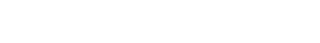1.2 Multi-channel variable step size

The MIMO filter-x LMS algorithm is the most widely used noise control algorithm. The system block diagram is shown in Figure 1. Suppose there are I reference sensors, J secondary speakers, and K error sensors in the system. The IJ adaptive filters are transversal filters whose length is L, and the filter weight coefficients are uniformly expressed as W(z) in vector form. Hp(z) represents the transfer function of the IK primary paths, Hs(z) represents the transfer function of the JK secondary paths, and Hs`(z) is the estimated value of the transfer function of the secondary paths. The primary and secondary paths are equivalent to FIR filters, assuming their lengths are Lp and Ls, respectively.

Let xi(n) be the input signal of the ith reference sensor at the nth time, which is called the ith reference signal, that is, xi(n)=[xi(n), xi(n-1),…,xi(n-L+1)]T; yj(n) is the output signal of the jth controller at the nth time, expressed as y(n) = [y1(n), y2(n),…, yJ(n)]T; dk(n) is the expected signal at the nth time at the kth error sensor, expressed as d(n) = [d1(n), d2(n),…, dK(n)]T; ek(n) is the error term signal at the n-th time here, expressed as e(n) = [e1(n), e2(n),…, eK(n)]T.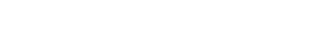Then the output signal of the jth speaker is sj(n) = yj(n)×Hs(n).The error signal vector can be written asIn the formula: Hs(n) is the K×J order sub-path impulse response matrix, and the (k, j)th element is hskj(n); r(n) is the J×KLs order filter-x signal matrix, which (j , k) element is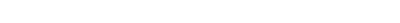The objective function of the multi-channel adaptive active control system is set as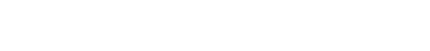Similar to the commonly used FxLMS algorithm, using the principle of the steepest descent method, the iterative formula of the controller weight coefficient can be derived as:

w(n +1) = w(n)−2μr(n)e(n) (10)

The whole algorithm expression is summarized as formulas (11)~(13)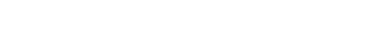The signal received by the kth error sensor is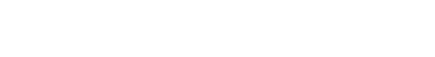Iterative formula of weight coefficients from the ith reference sensor to the jth controller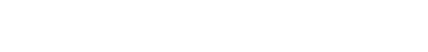in the formula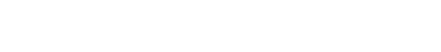where μ is the convergence step size, which can be obtained from equation (5). Then this algorithm is named variable-step-size multi-channel filtering x least mean square algorithm (VM-FxLMS).

2 Simulation experiment

2.1 Single-channel algorithm performance

The ANC system model is established in MATLAB, and the signal simulates the noise of the exhaust fan. Fan noise is composed of rotating noise and flocculation noise. The rotating noise is represented by a sinusoidal signal with a frequency of about 500 Hz, and the flocculation noise is represented by white noise with a cut-off frequency of 4 kHz. Add a low-pass filter to the system to filter out noise above 4 kHz.

The following will use the FxLMS algorithm, the normalized NLMS algorithm, the SVSLMS algorithm and the single VM-FxLMS algorithm proposed in this paper to test and compare the simulated noise. The initial convergence step μ of the three algorithms is set to 0.01. The simulation test results are shown in Figure 2.In the initial convergence state, it can be seen from Figure 2 a) and b) that the convergence speed of the variable-step algorithm has obvious advantages over the fixed-step algorithm. The single VM-FxLMS algorithm proposed in this paper has a fast convergence speed, and the algorithm can further converge when other algorithms tend to be stable. In the steady-state stage, it can be seen from Figure 2 c) that the single-VM-FxLMS algorithm has the lowest steady-state error and is stable without obvious fluctuations. It can be seen from the simulation results that the algorithm proposed in this paper is better than the traditional fixed-step FxLMS algorithm and some other variable-step algorithms.

2.2 Implementation of multi-channel area noise reduction

A 4 m × 4 m area is simulated in MATLAB, and multiple noise sources are set in the area. The noise components are the same as the noise sources set in Section 2.1, and more interference is added. The initial convergence step μ is set to 0.01, and the multi-channel ANC system is set to 16 channels. Figure 3 shows the noise simulation graphs before and after noise reduction in this area.As shown in Figure 3 a), the average noise in this area is about 50 dB, and the peak noise is about 60 dB. The simulation diagram after noise reduction is shown in Figure 3 b). Except for individual points, the noise in the entire area has been significantly reduced, and the average residual noise is about 25 dB. After noise reduction, the noise is reduced by about 25 dB compared to before noise reduction, and there is an increase in noise in individual areas. This simulation experiment shows that the multi-channel ANC system proposed in this paper has a very good control effect on regional noise reduction.

3 Conclusion

The multi-channel noise active control method based on variable step size proposed in this paper uses the variation of the reference signal as the input signal of the variable step size function, which can improve the non-zero offset of the algorithm. Compared with other commonly used control algorithms, the convergence There are obvious advantages in speed and convergence effect. Then the variable step size algorithm is extended to the multi-channel active control algorithm. For the simulation of noise control in a large area, the multi-channel ANC system shows a very good noise reduction effect. At the same time, the computational load of the multi-channel ANC system is a focus that needs further research, which is also the focus of future research work.